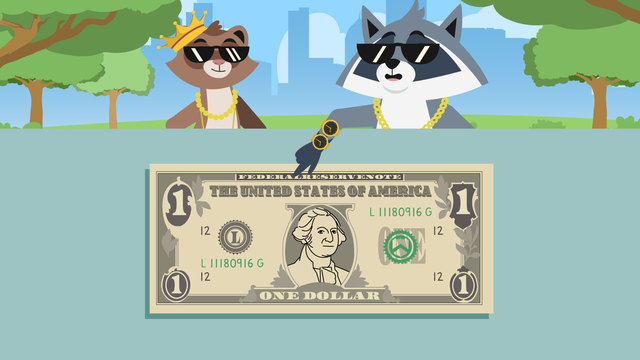# The Dollar (plus Dollar Notation)Rating

Ø 5.0 / 1 ratings

The authorsTeam Digital

## Basics on the topicThe Dollar (plus Dollar Notation)

### In This Video

Zuri and Freddie are learning all about the dollar, so they can spend the money they have. In the end, they spend a little too much money, and are disappointed when they can only afford one ice cream… but they have a creative solution!

### Teaching the Dollar 2nd Grade

There is a special symbol used to represent the dollar. It is an S with a vertical line through it, like in the image below.There are six commonly used dollar bills in the US, and these are one dollar, five dollars, ten dollars, twenty dollars, fifty dollars, and one hundred dollars!To identify the value of a dollar bill, you can look at the number on the note, or the word form of the number on the note. In the example below, you can see where you might find these on a dollar bill. Every bill has the number form or word form of the value on it.### The Dollar - Summary

There are six commonly used dollar bills; one dollar, five dollars, ten dollars, twenty dollars, fifty dollars, and one hundred dollars. They all have the value in number or word form on them, so you know the value of the bill. Below you will find a dollar worksheet for practice.

### TranscriptThe Dollar (plus Dollar Notation)

"Zuri, you won't believe what I found out." "What, Freddie?" "We can use these to buy ice cream!" "What? With this paper?" Let's help Zuri and Freddie learn all about the dollar. The dollar is a form of paper currency, or money, that is used in America. Money in the United States is represented by dollars and cents. There is a special symbol, or notation, we use when writing money in dollars, and it looks like this. We know what the dollar notation looks like, but how do we write it? First, start by writing an S. Then, put a vertical line down the middle. Now you have seen how to write the dollar notation, lets see what dollar bills there are! There are six commonly used dollar bills in the US. The one dollar bill, the five dollar bill, the ten dollar bill, the twenty dollar bill, the fifty dollar bill, and the hundred dollar bill! You can identify the value of a dollar bill by looking at the number on the bill, or the word form of the number on the bill! Before Zuri and Freddie can spend some of their savings, let's practice identifying some dollar bills and writing their value! Here is our first dollar bill. What is the value of this? We can see ten in number form here and also in word form here, so the value is ten dollars! How do we write ten dollars? First write the dollar notation, then write a ten after it. We can also write it with a period followed by two zero's after it, where the numbers after the period would represent cents. "Okay, so that's ten dollars!" "What about this one?" What is the value of this dollar bill? We can see one in number form here, and also in word form here so the value is one dollar! How do we write one dollar? First write the dollar notation, then write a one after it. We can also write it like this. Zuri and Freddie now understand the dollar. While they head off to spend some money, let's review! Remember, the dollar is a form of paper currency, or money, that is used in America. We can write money in dollars by writing the dollar notation, or symbol, followed by a value. There are six commonly used dollars bills, which include the one dollar bill, the five dollar bill, the ten dollar bill, the twenty dollar bill, the fifty dollar bill, and the hundred dollar bill! "I'm glad we stopped for some new stuff before we get ice cream, Freddie, we look so cool" "Oh man, we only have enough left for one ice cream cone!" "Next time, let's be more careful with our money!"